• javaSE阶段练习题，包含选择，填空，问答等。覆盖了java基础常见知识点及问答题
• ## javaSE练习题

千次阅读 2018-08-03 20:43:06
考察了equals方法。MyClass类没有复写Object类中的equals方法，即此equals方法是比较两个对象的值是否相等。由于m1和m2是两个对象，对应的值则是他们的内存地址，所以equals方法返回false。 25.运行以下的...
1.在JAVA中，下列哪些说法是正确的( AC )
A.java源文件的扩展名为.java
B.写好的java源程序可以直接运行  //不编译就想运行？？？
C.编写的源程序必须先编译后才能运行
D.程序员可以读懂扩展名为.class的文件  //字节码文件，不可能读懂的

2.下列标识符哪个是合法的( BD )
A.class
B.美元符号abc
C.1234
D._name
变量名首字母是英文字母、\$或下划线开头，可以由字母、数字或美元符号组成。

3.下面哪些赋值语句是正确的?(ABD)
A. long test = 12;
B. float f = -41.2f;
C. int other = (int) true;//布尔型不能强转成整型
D. double d = 12345678;
E. byte b = 128;   //byte范围为：-128~127

4.在Java中，下列语句不能通过编译的有( BD)
A. String s= “join”+ 3;
B. int a= “join”+3;//错误，字符串类型不能转换成整型
C. int a= ‘a’+5;
D. float f=5+5.5;//错，小数点默认类型为double类型，转换成float类型需要进行强转

6.下列代码运行的结果是(  C )
public static void main(String[] args) {
int x = 4;
int y = 5;
if(x++>4 & ++y>5) {
x++;
}
System.out.println(“x=” + x +”,y=” + y);
}
A. x=4,y=5
B. x=4,y=6
C. x=5,y=6
D. x=6,y=6
x++,先用后加，++x先加后用。

7.尝试运行下面的代码会发生什么?( B )
public class Test {
public static void main(String[] args) {
boolean m = true;
if (m = false) {
System.out.println(“true”);
} else {
System.out.println(“false”);
}
}
}
A.输出字符串”true”
B.输出字符串”false”
C.编译时错误
D.运行时错误

8.编译并运行以下代码将发生什么? ( E )
public static void main(String args[]){
char digit = ‘a’;
for (int i = 0; i < 10; i++){
switch (digit)
{
case ‘x’ :
{
int j = 0;
System.out.println(j);
}
default :
{
int j = 100;
System.out.println(j);
}
}
}
int i = j;
System.out.println(i);
}
A.输出11次100
B.输出10次100，然后抛出运行期异常
C.编译错误，因为变量i不能在main()方法中被声明2次
D.编译错误，因为变量j不能在main()方法中被声明2次
E.以上都不对

class Demo
{
public static int fun(int c)
{
return c+=2;
}
public static void main(String[] args)
{
int temp = fun(2);
System.out.println(temp);
}
}
打印结果是（B）
A. 2
B. 4
C. 6
D. 8

10.下面哪些函数是public void aMethod(){…}的重载函数?( BD )
A. void aMethod( ){…}
B. public int aMethod(int a, float b){…}
C. public void aMethod (){…}
D. public float aMethod (int m){…}
重载就记住一句：方法名相同，参数序列不同，跟返回值无关

11.在java中，关于数组描述正确的是( BD )
A.数组中的索引下标从1开始//从0开始
B.存储在数组当中的数据都属于同一数据类型//正确
C.通过数组名.length()能获得数组的长度//错误，length是获取数组的长度
D.数组的最大索引下标是数组的长度减1//正确

12.下面程序的输出结果是什么(D )
int[] arr = new int;
System.out.println(arr);
A.编译不通过
B.运行时出错
C.输出null
D.输出0

13.下面哪个语句正确地声明一个整型的二维数组( CD )
A. int a[][] = new int[][];
B. int b = new int[][];
C. int c[][] = new int;
D. int []d[] = new int;

14.以下代码输出是( D )
}class Demo {
public static void main(String[] args) {
int i = 0;
int sum = 0;
while (i <= 10) {//10以内
i++;
if (i % 2 != 0)//偶数
continue;
sum += i;//求和
}
System.out.println(sum);
}

A. 55
B. 45
C. 35
D. 30
计算10以内的偶数的和。

和下面代码能完成相同的选项是( B )
class Demo {
public static void main(String[] args) {
int i = 1;
int sum = 0;
while (i <= 100) {//100以内
if (i % 2 == 0) {//偶数
sum = sum + i;//求和
}
i++;
}
System.out.println(sum);
}
}
A. for (int x =1; x<=100;x++){sum=sum+x;}//求1到100的和
B. for (int x =0; x<=100;x+=2){sum=sum+x;}//求1到100之间偶数的和
C. for (int x =1; x<=100;x+=2){sum=sum+x;}//求1到100之间奇数的和
D.上述全对

16.下列有关类、对象和实例的叙述，正确的是哪一项?( D )
A.类就是对象，对象就是类，实例是对象的另一个名称，三者没有差别
B.对象是类的抽象，类是对象的具体化，实例是对象的另一个名称
C.类是对象的抽象，对象是类的具体化，实例是类的另一个名称
D.类是对象的抽象，对象是类的具体化，实例是对象的另一个名称//正确

17.下面关于java中包的说法正确的是(ACD )
A.在java中可以使用import语句导入包
B.在java中可以使用package语句导入包
C.位于同一个包中的类，不需要导包就可以直接访问
D.不同的包中可以出现类名相同的类

18.下列有关抽象类的叙述正确的是哪项? (C)
A.抽象类中一定含有抽象方法//不一定，普通类也可以使用abstract变成抽象类
B.抽象类既能被实例化也能被继承//抽象类不能实例化，可以被继承
C.抽象类的声明必须包含abstract关键字//正确
D.抽象类中不能有构造方法//抽象类可以有构造方法，接口不能有构造方法

下列有关接口的叙述错误的是哪项?( D )
A.接口中只能包含抽象方法和常量
B.一个类可以实现多个接口
C.类实现接口时必须实现其中的方法
D.接口不能被继承//接口能被继承，也能被实现

20.运行以下代码片段，输出结果是?( D )
class X {
Y b = new Y();
X() {
System.out.print(“X”);
}
}
class Y {
Y() {
System.out.print(“Y”);
}
}
public class Z extends X {
Y y = new Y();
Z() {
System.out.print(“Z”);
}
public static void main(String[] args) {
new Z();
}
}
A. Z
B. YZ
C. XYZ
D. YXYZ

main函数中，new Z(),访问类 Z，由于 Z 继承了 X,又转向去访问类X，类X里面又创建了类Y的对象，又转向了Y，所以第一步是打印Y，再回到类X中构造函数，打印X，再返回到类Z中，由于类Z中也有类Y 的对象，又要返回类Y调用一次类Y 的构造函数，打印Y，最后回到类Z中，打印Z。
21. 下列关于类的继承的描述，正确的有( BD )
A. 一个类可以同时继承多个父类//java不支持多继承
B. 一个类可以具有多个子类
C. 子类会自动拥有父类所有的方法//构造方法不能被继承
D. 一个类继承另一个类需要使用extends关键字

22.下列选项中关于java中this关键字的说法错误的有( BD )
A. this关键字是本类对象对自身的引用
//错误，应该是当前类对象对自身的引用
B. this关键字可以用于引用当前类以外其他类型的对象//错误，应该是当前类
C. this可用于构造函数中，调用类本身重载的构造函数，但是必须写在首行//正确
D. this可用于静态方法中//错误。静态方法与对象无关，this与对象有关

23.下列关于构造函数的说法正确的是( ABD )
A.方法名必须与类名相同
B.使用new关键字创建对象时，java虚拟机会自动调用构造函数
C.我们在定义一个类时，必须要声明至少一个构造函数 //没有声明构造函数是，会有一个默认的无参构造函数
D.构造函数中不能使用return语句

24.编译并运行以下代码将发生什么?( D )
class MyClass {
int x;
MyClass(int i) {
x = i;
}
public static void main(String args[]) {
MyClass m1 = new MyClass(100);
MyClass m2 = new MyClass(100);
if (m1.equals(m2)) {
System.out.println(“Both areequal”);
} else {
System.out.println(“Both are notequal”);
}
}
}
A.代码编译时报出错误提示信息“equals()方法未定义”
B.编译通过，抛出运行期异常.
C.输出Both are equal.
D.输出Both are not equal

本题考察了equals方法。MyClass类没有复写Object类中的equals方法，即此equals方法是比较两个对象的值是否相等。由于m1和m2是两个对象，对应的值则是他们的内存地址，所以equals方法返回false。

25.运行以下的main()方法，结果是?( AC )
1 public static void main(String[] args)
2 {
3 String myString;
4 int x = 100;
5
6 if (x < 100) myString = “x isless than 100”;
7 if (x > 100) myString = “x isgreater than 100”;
8 System.out.println(myString.length());
9 }
A.编译时报出错误提示信息“变量myString没有被初始化”
B.编译通过
C.编译未能通过。但如果变量myString在第8行前的代码中被初始化，代码可以编译通过，运行时可以输出字符串myString的长度
D.以上都不对
局部变量没有被初始化，编译不能通过；初始化后能正常执行。

26.有如下代码，请问哪些是正确的?( ACD )
class ClassA{}
class ClassB extends ClassA{}
class ClassC extends ClassA{}
以及
ClassA p0 = new ClassA();
ClassB p1 = new ClassB();
ClassC p2 = new ClassC();
ClassA p3 = new ClassB();
ClassA p4 = new ClassC();
A.p0 = p1;//正确，类型自动向上提升
B.p1 = p2;//错误，类型转换异常
C.p1 = (ClassB)p3;//正确，类型强制向下转型
D.p2 = (ClassC)p4;//正确，类型强制向下转型

A.Java中只支持单继承,一个类只能继承一个类,但是可以有多个子类
B.一个类如果没有自己写无参构造方法,那么子类将无法继承//错误，如果自己没有写构造方法，会有默认的隐式的无参构造方法
C.子类可以当父类用,父类不可以当子类用//父类强转之后也可当之类使用
D.子类重写父类方法时访问权限不能更低

28.编译并运行以下代码的输出结果是?( A )
String s1 = new String(“amit”);
System.out.println(s1.replace(‘m’,’r’));
System.out.println(s1);
String s3 = “arit”;
String s4 = “arit”;
String s2 = s1.replace(‘m’,’r’);
System.out.println(s2 == s3);
System.out.println(s3 == s4);
A. arit
amit
false
true
B. arit
arit
false
true
C. amit
amit
false
true
D. arit
amit
true
true

29.下列哪些代码可以顺利通过编译和运行? ( ABD )
A.Integer i = new Integer(‘A’);
B.Integer i = new Integer(“7”);
C.Character c = newCharacter(“A”);
D.Boolean b = new Boolean(null);

30.在Java中，如果要在字符串类型对象s=”java”中，得到字符’v’出现的位置，可使用下面的那个方法?( C )
A. s.matches(‘v’);
B. s.charAt(‘v’);
C. s.indexOf(‘v’)；
D. s.substring(‘v’);

A. ArrayList集合底层是数组实现，该集合线程不安全
B. Vector集合元素的存放是无序的
D.这三个集合都是List接口的实现类

32.下面程序的输出结果是什么( D )
Set set= new HashSet();
System.out.println(set.size());
A.编译不通过
B.运行时出错
C.输出3
D.输出2

B. removeFirst()
C. remove(Object o)

34.下列哪些集合属于Collection体系的子类( BD )
A. TreeMap
B. ArrayList
C. Hashtable
D. HashSet

35.下面有关Java异常处理的说法错误的是( A )
A.一个try块只能有一条catch语句//可以有多条Catch语句
B.一个try块中可以不使用catch语句
C. catch块不能单独使用，必须始终与try块在一起
D. finally块不能单独使用，必须始终与try块在一起

36.下列关键字能和try语句一起使用的是( CD )
A. final
B. finalize
C. finally
D. catch
final，关键字，被修饰成员变量不可变;finalize,垃圾收集器执行时会调用被回收对象的finalize方法；finally，异常处理部分必须被执行的部分；catch，异常处理时必须和try一起使用。

37.下面程序的运行结果是( D )
FileOutputStream fos = newFileOutputStream(“c:\demo.txt”);
fos.write(“abc”);
fos.close();
A.在C盘创建文件demo.txt,但文件是空的
B.在C盘创建文件demo.txt,并写入数据abc
C.将C盘已有的文件demo.txt中追加写入abc
D.编译失败
编译失败。FileOutputStream为字节输出流，它的write方法不能直接传入字符串，应该利用getBytes()方法把字符串转换成字节数组。

38.下列关于Java中文件输入输出的说法正确的是( AC )
A.在Java中，文件的输入输出功能是通过流来实现的
B.如果要把数据写入到一个文件中，需要创建一个输入流对象  //输出流对象
C.字符流在某些方面比如汉字的处理，比字节流更方便

39.请看如下代码：
BufferedWriter bw = new BufferedWriter(newFileWriter(“d:\b.txt”));
String line = null;
while ((line = br.readLine()) != null) {//读取a.txt文件的内容
bw.write(line);//将读取到的内容写入到b.txt
bw.newLine();
bw.flush();
}
bw.close();
br.close();
它表达的意思是?( A )
A.把c盘目录下的a.txt文件内容复制到d盘目录下的b.txt
B.把d盘目录下的b.txt文件内容复制到c盘目录下的a.txt
C.读取c盘目录下a.txt文件，输出在控制台
D.把控制台的内容写入到d盘目录下的b.txt文件中

40.关于泛型的说法正确的是( ABC )
A.泛型是JDK1.5出现的新特性
B.泛型是一种安全机制
C.使用泛型避免了强制类型转换
D.使用泛型必须进行强制类型转换

41.关于方法的递归算法，说法正确的是( AB )
A.递归就是方法自己调用自己
B.递归的次数不能过大，否则会导致栈内存溢出
C.使用递归算法，方法必须有返回值
D.构造方法不可以使用递归算法

42、. 在当前的java  实现中，每个文件编译之后就是一个以（B）为后缀的文件
A.java
B.class
C. doc
D. exe
43.下列属于三目(  B)  运算符的是：
A. +
B. ？：
C.&&
D. |
44.在 java 语句中,运算符&&实现(B)
A. 逻辑或
B. 逻辑与
C. 逻辑非
D. 逻辑相等
45.byte变量的取值范围是 (B)
A.  0 - 65535
B.  -128 – 127
C. -256 – 255
D. 0 - 32767
二、简答题
1.抽象类和接口的区别?
（1）抽象类和接口都不能直接实例化，如果要实例化，抽象类变量必须指向实现所有抽象方法的子类对象，接口变量必须指向实现所有接口方法的类对象。
（2）抽象类要被子类继承，接口要被类实现。
（3）接口只能做方法申明，抽象类中可以做方法申明，也可以做方法实现
（4）接口里定义的变量只能是公共的静态的常量，抽象类中的变量是普通变量。
（5）抽象类里的抽象方法必须全部被子类所实现，如果子类不能全部实现父类抽象方法，那么该子类只能是抽象类。同样，一个实现接口的时候，如不能全部实现接口方法，那么该类也只能为抽象类。
（6）抽象方法只能申明，不能实现，接口是设计的结果 ，抽象类是重构的结果
（7）抽象类里可以没有抽象方法
（8）如果一个类里有抽象方法，那么这个类只能是抽象类
（9）抽象方法要被实现，所以不能是静态的，也不能是私有的。
（10）接口可继承接口，并可多继承接口，但类只能单根继承。
2.ArrayList 、Vector 、LinkedList 的区别，HashMap 和 Hashtable 的区别?
ArrayList和Vector都是使用数组方式存储数据，此数组元素数大于实际存储的数据以便增加和插入元素，它们都允许直接按序号索引元素，查询速度快，
但是插入元素要涉及数组元素移动等内存操作，所以索引数据快而插入数据慢
Vector是同步处理,性能较低；ArrayList是使用异步处理，性能高。
一般情况都用ArrayList，ArrayList占据内存小.
HashMap 和 Hashtable 的区别:
两者最主要的区别在于Hashtable是线程安全，而HashMap则非线程安全;HashMap可以使用null作为key，而Hashtable则不允许null作为key;HashMap是对Map接口的实现，HashTable实现了Map接口和Dictionary抽象类;
展开全文• JavaSE练习题笔记整理 1.键盘录入判断奇偶数： 将操作步骤抽取，做成一个方法调用 public static void main(String[] args) { //调用getSum()方法 getSum(); } public static void getSum(){ //创建键盘输入对象 ...
JavaSE练习题笔记整理
1.键盘录入判断奇偶数：
将操作步骤抽取，做成一个方法调用
public static void main(String[] args) {
//调用getSum()方法
getSum();
}
public static void getSum(){
//创建键盘输入对象
Scanner sc = new Scanner(System.in);
//给出提示
System.out.println(“请输入第一个整数：”);
//使用变量来接收用户录入的数据
int num = sc.nextInt();
//使用if…else分支语句结构对录入的数据进行判断
if (num % 2 == 0) {
System.out.println(“您输入的数字” + num + “是一个偶数”);
} else {
System.out.println(“您输入的数字” + num + “是一个奇数”);
}
}
2.switch语句判断春夏秋冬：switch具有穿透性
public static void main(String[] args) {
//创建键盘录入对象
Scanner sc = new Scanner(System.in);
System.out.println(“请输入您的月份，系统会自动判断月份,用户放心输入：”);
int num = sc.nextInt();
//switch语句判断春夏秋冬：switch具有穿透性
switch(num){
case 1:
case 2:
case 3:
System.out.println(“该月份为春天”);
break;
case 4:
case 5:
case 6:
System.out.println(“该月份为夏天”);
break;
case 7:
case 8:
case 9:
System.out.println(“该月份为秋天”);
break;
case 10:
case 11:
case 12:
System.out.println(“该月份为冬天”);
break;
default:
System.out.println(“您输入的月份有误请重新输入”);
System.exit(0);  //停止JVM运行
}
}
3.判断一张纸（厚度0.1）折叠多少次可以达到珠穆朗玛峰的高度（8844430m）
public static void main(String[] args) {
    //调用getHigth()方法
getHigth();
}
public static void getHigth(){
//定义纸张的厚度
double paper = 0.1;
//定义一个变量统计最终折叠的次数（相当于计数器）
int count = 0;
//可以使用循环来完成，因为不知道要折叠多少次
while(paper<=8844430){
//纸张加倍
paper *= 2;
//计数器加1
count++;
}
//输出折叠的次数
System.out.println("需要折叠："+count+"次,就可以达到珠穆朗玛峰的高度！");
}
4.数组反向输出
public static void main(String[] args) {
//定义一个int类型静态初始化数组
int[] arr = {12,56,75,86,24,32,27,64};
//数组反转前的遍历
System.out.println("数组反转前");
System.out.print("[");
for (int i = 0; i < arr.length; i++) {
//判断最后一个元素，在后面加上】符号
if(i==arr.length-1){
System.out.println(arr[i]+"]");
}else {
System.out.print(arr[i] + ", ");
}
}
//数组反转后 ： 定义一个变量来接收元素之间的转换
for(int i=0,j=arr.length-1;i<=j;i++,j--){
int temp = arr[i];
arr[i] = arr[j];
arr[j] = temp;
}
System.out.println("数组反转后");
//数组反转后的遍历
System.out.print("[");
for (int i = 0; i < arr.length; i++) {
//判断最后一个元素，在后面加上】符号
if(i==arr.length-1){
System.out.println(arr[i]+"]");
}else {
System.out.print(arr[i] + ", ");
}
}
}



展开全文• 打印菱形 可以用线性代数解决 八大排序 冒泡排序 相邻元素两两比较，大的往后放，第一次完毕，最大值出现在了最大索引处。同理，其他的元素就可以排好。 public static void bubbleSor...

打印菱形      可以用线性代数解决

八大排序
冒泡排序             相邻元素两两比较，大的往后放，第一次完毕，最大值出现在了最大索引处。同理，其他的元素就可以排好。                          public static void bubbleSort(int[] arr) {                 for(int x=0; x<arr.length-1; x++) {                     for(int y=0; y<arr.length-1-x; y++) {                         if(arr[y] > arr[y+1]) {                             int temp = arr[y];                             arr[y] = arr[y+1];                             arr[y+1] = temp;                         }                     }                 }             }
选择排序             把0索引的元素，和索引1以后的元素都进行比较，第一次完毕，最小值出现在了0索引。同理，其他的元素就可以排好。                          public static void selectSort(int[] arr) {                 for(int x=0; x<arr.length-1; x++) {                     for(int y=x+1; y<arr.length; y++) {                         if(arr[y] < arr[x]) {                             int temp = arr[x];                             arr[x] = arr[y];                             arr[y] = temp;                         }                     }                 }             }
二分查找(折半查找)             针对数组有序的情况(千万不要先排序，在查找)                          public static int binarySearch(int[] arr,int value) {                 int min = 0;                 int max = arr.length-1;                 int mid = (min+max)/2;                                  while(arr[mid] != value) {                     if(arr[mid] > value) {                         max = mid - 1;                     }else if(arr[mid] < value) {                         min = mid + 1;                     }                                          if(min > max) {                         return -1;                     }                                          mid = (min+max)/2;                 }                                  return mid;             }
汉诺塔
Java对象的假克隆       Java对象的浅克隆      Java对象的深克隆
序列化与对象克隆       深克隆效率的比较

转载于:https://my.oschina.net/u/3611296/blog/1478552
展开全文• Home22 (倒排一个字符串)编写-一个程序，提示用户输人一个字符串，然后以反序显示该字符串。 import java.util.Scanner; class Home{ public static void main(String[] args){ Scanner scanner =new Scanner...
Home22
(倒排一个字符串)编写-一个程序，提示用户输人一个字符串，然后以反序显示该字符串。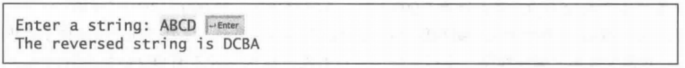import java.util.Scanner;
class Home{
public static void main(String[] args){
Scanner scanner =new Scanner(System.in);
System.out.print("请输入字符串");
String a=scanner.nextLine();
String b="";
char c[]=a.toCharArray();
for(int i=c.length-1;i>=0;i--){
b=b+c[i];
}
System.out.print(b);
}
}

Home23
(对大写字母计数)编写一个程序，提示用户输入一个字符串，然后显示该字符串中大写字母的数目。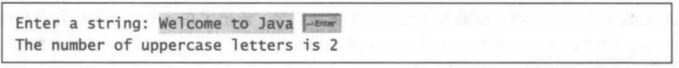import java.util.Scanner;
class Home23{
public static void main(String[] args) {
Scanner scanner =new Scanner(System.in);
System.out.print("Enter a string:");
String str=scanner.nextLine();
int sum=0;
char c[]=str.toCharArray();
for(int i=0;i<str.length();i++){
if(c[i]<='Z'&&c[i]>='A'){
sum++;
}
}
System.out.println("the number is uppercase letter is "+sum);
}
}

Home24
(反向显示一个整数)使用下面的方法头编写方法，反向显示一个整数:
public static void reverse(int number)
例如: reverse(3456) 返回6543。编写一个测试程序，提示用户输入一个整数，然后显示它的反向数。
import java.util.Scanner;
class Home24{
public static long sum=0;
public static void main(String[] args) {
Scanner scanner =new Scanner(System.in);
System.out.print("Enter a number:");
long n=scanner.nextLong();
reverse(n);
System.out.println(sum);
}
public static void reverse(long n){
while(true){
sum=sum*10+n%10;
n/=10;
if(n==0){
break;
}
}
}
}

Home25
(指定字符的出现次数)使用下面的方法头编写-一个方法，找到一个字符串中指定字符的出现次数。
public static int count(String str, char a)
例如，count(“Welcome”,‘e’) 返回2.编写一个测试程序，提示用户输人一个字符串以及一个字符，显示该字符在字符串中出现的次数。
import java.util.Scanner;
class Home25{
public static void main(String[] args){
Scanner scanner=new Scanner(System.in);
System.out.print("输入字符串:");
String str=scanner.nextLine();
System.out.print("输入查找元素:");
String ch=scanner.next();
int num=count(str,ch);
System.out.println(ch+"出现次数为"+num);
}
public static int count(String str,String ch){

int num=0;
while(str.indexOf(ch)!=-1){
str=str.substring(str.indexOf(ch)+1);
System.out.println(str.indexOf(ch));
num++;
}
return num;

/*
这里为另外一种方法
int num=0;
for(int i=0;i<str.length();i++){
if((str.charAt(i)+"").equals(ch)){
num++;
}
}
return num;
*/
}
}

Home26
(回文素数)回文素数是指一个数同时为素数和回文数。例如: 131 是一个素数，同时也是一个回文素数。数字313和757也是如此。编写程序，显示前100个回文素数。每行显示10个数并且准确对齐，数字中间用空格隔开。如下所示:
2 3 5 7 11 101 131 151 181 191
313 353 373 383 727 757 787 797 919 929
import java.util.Scanner;
class Home26{
public static void main(String[] args) {
//1.数字初始化
int num=initDate();
//2.判断该数是不是回文素数
if(isHWSS(num)){
System.out.println("yes");
}else{
System.out.println("no");
}
}
public static int initDate(){
Scanner scanner =new Scanner(System.in);
System.out.print("enter a number:");
return scanner.nextInt();
}public static boolean isHWSS(int num){
//3.判断是否满足回文和素数
return isHuiWen(num)&&isSuShu(num);
}
public static boolean isHuiWen(int num){
//4.计算翻转数字
int a=reverse(num);
return a==num;
}
public static int reverse(int num){
int sum=0;
while(true){
sum=sum*10+num%10;
num/=10;
if(num==0){
break;
}
}
return sum;
}
public static boolean isSuShu(int num){
//5.判断素数
boolean flag=true;
for(int i=2;i<=num/2;i++){
if(num%i==0){
flag=false;
break;
}
}
return flag;
}
}

Home27
(游戏:双骰儿赌博)掷双骰子游戏是赌场中非常流行的骰子游戏。编写程序，玩这个游戏的一个变种，如下所示:
  掷两个骰子。每个骰子有六个面，分别表示值1, 2, ... 6。检查这两个骰子的和。如果和为2、3或12 (称为掷骰子(craps),你就输了;如果和是7或者11 (称作自然( natural)),你就赢了;但如果和是其他数字(例如: 4、5、 6、8、9或者10),就确定了一个点。继续掷骰子，直到掷出一个7或者掷出和刚才相同的点数。如果掷出的是7,你就输了。如果掷出的点数和你前一次掷出的点数相同，你就赢了。

程序扮演一个独立的玩家。下面是一些运行示例。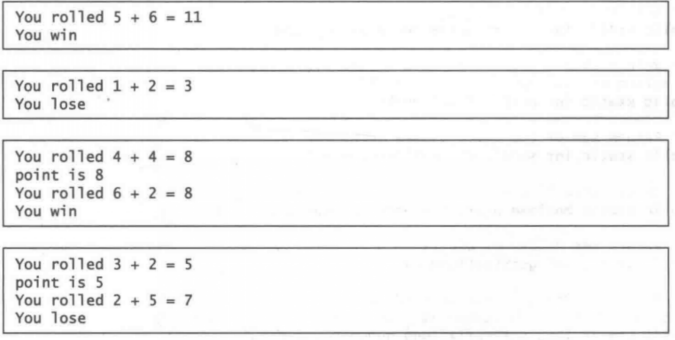import java.util.Random;
class Home27{
public static void main(String[] args) {
Random random=new Random();
//随机两个数模拟摇毂子
int a =random.nextInt(6)+1;
int b =random.nextInt(6)+1;
//int a=(int)(Math.random()*6+1);
//int b=(int)(Math.random()*6+1);
int c=a+b;
System.out.printf("You rolled %d + %d = %d \n",a,b,c);
if (c==2||c==3||c==12){//如果两数加起来是这三个值则输
System.out.println("you lose");
}else if(c==7||c==11){//如果两数加起来是这三个值则输
System.out.println("you win");
}else{
while(true){
//对这两个值进行重新赋值
a=(int)(Math.random()*6+1);
b=(int)(Math.random()*6+1);
int d=a+b;
System.out.printf("You rolled %d + %d = %d \n",a,b,d);
if(d==c){//如果和上一个值相同则赢
System.out.println("you win");
break;
}else if(d==7){//如果摇出来7则输
System.out.println("you lose");
break;
}else{
//摇出来其他值，将该值赋给c，进行下一轮循环
c=d;
}
}
}
}
}

Home28
(打印不同的数)编写-一个程序，读人10个数并且显示互不相同的数(即-一个数出现多次,但仅显示一次)。(提示，读人一个数，如果它是一个新数，则将它存储在数组中。如果该数已经在数组中，则忽略它。)输人之后，数组包含的都是不同的数。下面是这个程序的运行示例: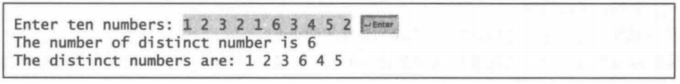Home32
(合并两个有序列表)编写下面的方法，将两个有序列表合并成-一个新的有序列表。
  public static int[] merge(int[] 1ist1, int[] 1ist2)

只进行1ist1. length+1ist2. 1ength次比较来实现该方法。编写-一个测试程序，提示用户输人两个有序列表，然后显示合并的列表。下面是- 一个运行示例。注意，输人的第-一个数字表示列表中元素的个数。该数字不是列表的一部分。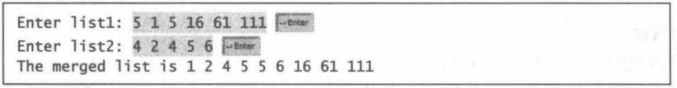import java.util.*;
class Home32{
/*
1.提示用户输入第一个数组长度和元素
2.提示用户输入第二个数组长度和元素
2.创建数组等于二者之和
4.有序倒到第三个
*/
public static void main(String[] args) {
int[] list1={1,3,5,7,9};
int[] list2={2,4,6,8,10};
int p1=0;
int p2=0;
int[] list3=new int[list1.length+list2.length];
for(int i=0;i<list3.length;i++){
if(p1>=list1.length){
list3[i]=list2[p2++];
}else if(p2>=list3.length){
list2[i]=list1[p1++];
}else if(list1[p1]<=list2[p2]){
list3[i]=list1[p1++];
}else{
list3[i]=list2[p2++];
}
}
System.out.print(Arrays.toString(list3));
}
}

(代数方面:两个矩阵相乘)编写两个矩阵相乘的方法。方法头如下:
public static double[][] multiplyMatrix(double[][] a, double[][] b)
为了使矩阵a能够和矩阵b相乘，矩阵a的列数必须与矩阵b的行数相同，并且两个矩阵的元素要具有相同或兼容的数据类型。假设矩阵c是相乘的结果，而a的列数是n,那么每个元素Cy就是anX by+axb.+…+agxbyo例如，对于两个3x3的矩阵a和b, c就有:这里的Cij=Ai1B1j+Ai2B2j+Ai3*B3j.
编写一个测试程序，提示用户输人两个3x3的矩阵，然后显示它们的乘积。下面是一个运行示例: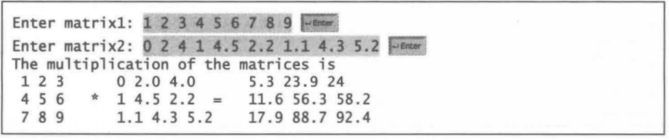class Home33{
public static void main(String[] args) {
double A[][]={
{1,2,3},
{4,5,6},
{7,8,9}
};
double B[][]={
{0,2.0,4.0},
{1,4.5,2.2},
{1.1,4.3,2.2}
};
double[][] C=new double[A.length][B.length];
for(int i=0;i<C.length;i++){
for(int j=0;j<C[i].length;j++){
C[i][j]=getSum(A,B,i,j);
System.out.print(C[i][j]+" ");
}
System.out.println();
}
}
public static double getSum(double[][] A,double[][] B,int i,int j){
double sum=0;
//Cij=Ai1*B1j+Ai2*B2j+Ai3*B3j
for(int k=0;k<B.length;k++){
sum+=A[i][k]*B[k][j];
}
return sum;
}
}



展开全文• 1.使用自己的话表述MVC。 MVC全名是Model View Controller，是模型(model)－视图(view)－控制器(controller)的缩写，一种软件设计典范，用一种业务逻辑、数据、界面显示分离的方法组织代码，将业务逻辑聚集到一个...
• 里面的练习题都是自己在平时生活中总结来的，希望大家能够用到！ 云盘 链接：https://pan.baidu.com/s/1OAZGWM9SphZPJ_0dk_z9vg 提取码：s74u ^_^! 顺便写了一些简单的if for的结构题 求递归 用Switch求随机...
• 第一 按步骤编写代码，效果如图所示： 编写步骤： 定义类 Test1 定义 main方法 控制台输出5行字符串类型常量值 控制台输出5行字符类型常量值 class Test1{ public static void ...java
• package com.javaTestDemo; import java.util.Scanner; public class JavaTest1 { public static void main(String[] args) { // 1. //countRabbit(); // 2. //countSuNumber();......
• 成员变量基础练习 第一 第二 第三 成员方法基础练习 第四： 第五： 第六： 第七： 第八： 第七章 基础练习 第一 第二 第三 第四 第五 递归练习（难） 第六 第七 ...java
• 第一 案例：在子线程中输出1-100之间的偶数，主线程输出1-100之间的奇数。 效果如下： package com.atguigu.test01; ​ public class Test01 { public static void main(String[] args) { new Thread(...java
• 工具包内包含：JavaSE基础阶段，从Java的概述到JavaSE阶段完结的所有JavaSE习题与答案、JavaSE所有思维导图和各种API文档。用以提供给大家下载、学习和训练。
• javaSE习题答案及解析 选择 执行代码 int i=6,j=8,k=10,m=7; if(i < j | m > ++k) k++; 后，k的值是多少 “|”运算符：不论运算符左侧为true还是false，右侧语句都会进行判断.理解这个就会很简单了 答案:......

# javase练习题java 订阅